## Recap

Last time, we used a set of arrows and vertices to represent a sequence of notes:

 such as the 'Cantus 1' line found in the first section of C T Padbrué's Synphonia in nuptias (for the marriage betwixt Steyn & van Napels) Your browser does not support the audio element. If the player doesn't work, there's some ogg vorbis over here.as a graph: reducible (eliminating uni-cycles and bi-cycles) to: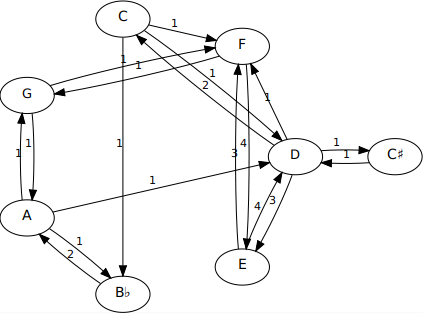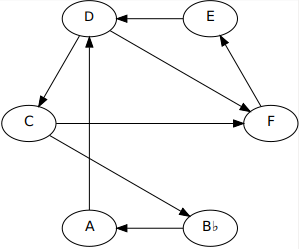whereupon we recovered the following three 'distillations' of the three lines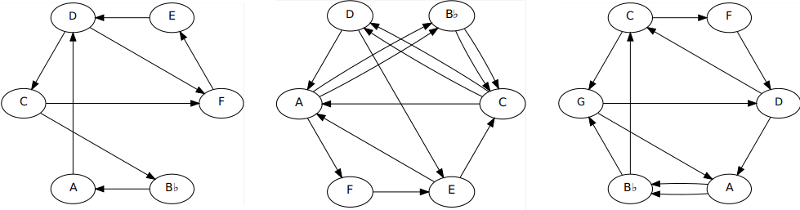Your browser does not support the audio element. We concocted a couple of examples using the reduced lines (as before, if the player doesn't work, there's some mp3 over here).

These directed graphs are known as quivers, consisting as they do of bunches of arrows, often many together (all facing the same direction) between vertex pairs since we've eliminated arrows which cancel each other out (i.e. all bi-cycles).

We wondered how many paths (if any) - consuming all arrows, exactly once - could be used to traverse each of these three graphs. It turns out (via nothing more sophisticated than brute force exhaustive searching) that for these three distillations there are (3, 130, 55) such paths. We show the three for cantus 1 and three typical ones for the other lines:

Cantus 1
(8 arrows)
Cantus 2
(13 arrows)
Bassus
(11 arrows)
C F E D C B♭ A D F
C B♭ A D C F E D F
C B♭ A D F E D C F
C D E C D A F E A B♭ C A B♭ C
D E C D A F E A B♭ C A B♭ C D
A F E A B♭ C A B♭ C D E C D A
G D C F D A B♭ C G A B♭ G
B♭ G D C G A B♭ C F D A B♭
D A B♭ C F D C G A B♭ G D

## Mutation Primer

The following describes an operation which - as someone said back in the 1990s when it was first being investigated - is elementary but complex.

We consider the idea of a quiver mutation as applied to these reduced melodic lines. It's necessary to perform these reductions before we mutate since the rules of mutation require it. The mutation is performed - reversibly - on a vertex of the quiver. In our case the vertex represents a musical pitch, but we don't change that pitch. Instead we alter its connections to its preceding and succeeding pitches by swapping their places and completing a circuit. Basically, the rules are - at the selected vertex:

1. Reverse all arrows entering and leaving the mutating vertex, M.
2. For every pair of vertices (P, S) now entering and exiting M (a 2-path from P(redecessor) to S(uccessor) via M), 'complete the circuit' PMS by adding a new arrow from S to P.
3. Maintain the 'no bi-cycle' constraint on the quiver by removing any newly introduced (by circuit completion) oppositely-directed arrow pairs.

We'll try to maintain a certain consistency of terminology here in distinguishing

• an arrow - a single directed edge (with one 'arrowhead') from one vertex to another, different one (no uni-cycles)
• a path - a trip from one vertex to another via arrows
Thus all arrows are paths (perforce of length 1) but not all paths are arrows. A path may start and finish at the same vertex - this is a cycle, or circuit. Thus all circuits are paths but not all paths are circuits. Note that quiver rules eliminate (and mutation rules maintain) all circuits which are also paths of length 2 (no bi-cycles).

Note that in this specific discussion we're referring to a path of length 2 passing through a mutating vertex as a 2-path. The two (non-mutating) vertices must be different otherwise we'd have a bi-cycle between it and the mutating vertex.

We illustrate a sequence of mutations - successively on vertices 1, 2, 3, 2 - in the following figure: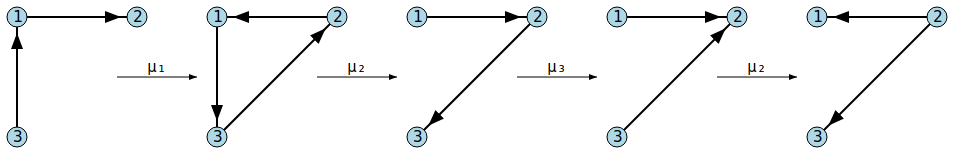The first mutation (μ₁) is on vertex labeled ① and reverses arrows ③→① and ①→②. As a consequence there's a 2-path from ②, via ①, to ③ and we 'complete the tri-cycle' by introducing a new arrow ③→②, thus producing the second quiver in the sequence. Since there were no opposing arrows from ② to ③, it remains.

The second mutation (μ₂) at vertex ② reverses arrows ③→② and ②→①. Consequently there's a 2-path from ① to ③ so we complete ②'s tri-cycle by adding a new arrow from ③ to ①. This time there's already an opposing arrow, ①→③. This would introduce a (forbidden) bi-cycle, so we annihilate the pair.

Note that at this stage (deftly avoiding the word 'point') a reapplication of μ₂ would exactly reverse what we have just done. All quiver mutations performed twice in succession at the same vertex are 'do nothings'.

The remaining mutations on vertices ③ and ② proceed as shown. The final mutation on ② turns that vertex from being a sink (no arrows leave it) into being a source (no arrows enter it).

Note that, in general, mutations on distinct nodes ⓜ and ⓝ do not commute, i.e. a quiver resulting from mutation by μₘ then μₙ is unlikely to be the same as the one resulting from μₙ then μₘ.

For this particular set of three vertices, 14 distinct configurations are possible with various applications of the three mutations: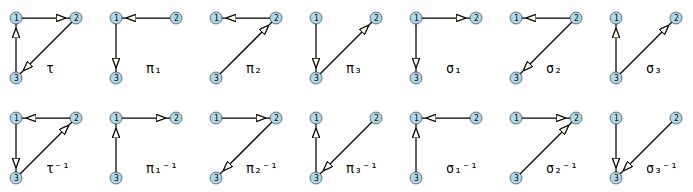In the above, we've labeled the configurations with a τ to suggest a triangle, πₙ to suggest a path through vertex n, and σₙ to suggest a source at vertex n. The inverses, e.g. τ⁻¹ and σₙ⁻¹ (a sink instead of a source) are essentially the same but with all arrows reversed. The following graph (edges bi-directional) shows all 21 ways of mutating between the 14 configurations.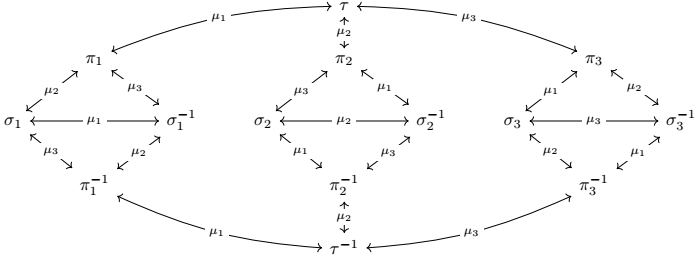The operations μ₁, μ₂ and μ₃ permute τ, π₁, π₂, π₃, σ₁, σ₂, σ₃, τ⁻¹, π₁⁻¹, π₂⁻¹, π₃⁻¹, σ₁⁻¹, σ₂⁻¹, σ₃⁻¹ without affecting the 'shape' of that graph (the vertex labels just - in this case - swap places seven pairs at a time). Thus we have a group which acts upon, and thereby represents the symmetries of, the structure held by these vertices. For example the first generator, μ₁, effects the seven simultaneous transpositions: (π₁,τ)(π₂,σ₂⁻¹)(π₃,σ₃)(σ₁,σ₁⁻¹)(σ₂,π₂⁻¹)(σ₃⁻¹,π₃⁻¹)(τ⁻¹,π₁⁻¹). And mutations μ₂ and μ₃ act - mutatis mutandis - similarly. The group generated is (C₂×C₂×C₂×C₂×C₂×C₂)⋉S₇, the semidirect product of the group (of order 64) formed from the direct product of 6 cyclic groups of order 2 with the group (of order 5040) of permutations of 7 symbols. This quiver group is of order 322560 = 7!(₈C₇)² = 7! × 64 = 2¹⁰×3²×5×7. The order of this group is 'coincidentally' the same as the number of ways 7 rooks may be placed on a standard 8×8 chessboard such that none may attack another.

## Musical Mutations

Needless to say, mutations on quivers with more than three vertices can get considerably more complicated. In general, there will be no limit to the number of configurations. If we consider the six possible mutations possible on the vertices of the above Cantus 1 distillation we can see, for example, that there are four 2-paths through the D vertex, i.e. ADC, ADF, EDC, EDF. All four of these 2-paths will be inverted by a mutation at D and will thereby engender four new arrows AC, AF, EC and EF; the last of these cancelling the existing arrow FE. Thus a quiver with - originally - 8 arrows becomes a quiver with 11. Mutations will always preserve the vertex set but, in general, the number of arrows will typically increase at each application as arrow generation will typically exceed any consequent cancellations.

Although it's possible to have finite quiver groups on six vertices, our trio of Padbrué 'compressings' - all, as it happens, with six vertices - are of infinite order. The six possible 'first mutations', for each quiver, are shown in the following diagram: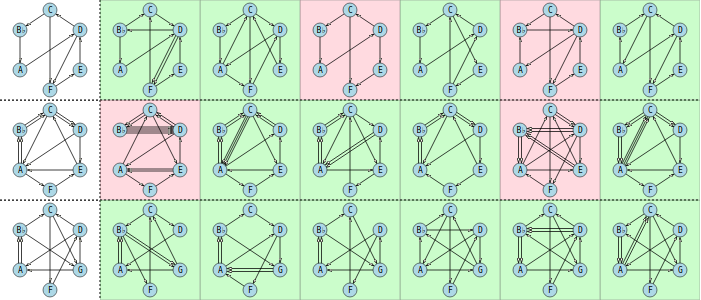The first column (with the uncoloured background) carries the reduction into quivers of each of the three lines in the Pavanne. The remaining six colums show, for each melody, the quivers resulting from mutations on each of the original quiver's six pitches in the order C, D, E, F, A and B♭ for canti 1 and 2 and in the order C, D, F, G, A and B♭ for the bass line. The green backgrounds denote quivers which may be fully traversed, in that it's possible to walk a path from some start vertex to some end vertex consuming each arrow exactly once. The pink backgrounds indicate quivers where this is impossible. This answers - in the negative - the question of its always being possible 'to find at least one path using all arrows in the graph' asked in the previous post.

Clearly a fully traversed path of n arrows will have n + 1 pitches in it from start to finish. Note that such paths are not cycles (Hamiltonian or otherwise) since no 'loop' is completed by terminating at the start node (one may certainly end at the same pitch one began on, but as each pitch may (in general) be visited many times in a traversal, such visits count as separate 'versions').

The following table shows the number of arrows in each of these (first) mutations and also - where possible - the number of full path traversals.

Cantus Iμ(C)μ(D)μ(E)μ(F)μ(A)μ(B♭)
arrows:810107899
# traversals:3614-3-5
Cantus IIμ(C)μ(D)μ(E)μ(F)μ(A)μ(B♭)
arrows:13161513121615
# traversals:130-31513010-315
Bassusμ(C)μ(D)μ(F)μ(G)μ(A)μ(B♭)
arrows:11111110111313
# traversals:5544445121234234

We've already shown above (and in the previous post) some melodies arising from full traversals of the unmutated quivers. We see that it's always possible to find a full traversal after one mutation at D for all lines (e.g. CDACB♭AFDECF for cantus 1, B♭CEDCADCAFEAB♭CAB♭ for cantus 2, and GADFAB♭GAB♭CDG for the bass line. Mutations at F for both canti provide melodies FCEFDCB♭AD and ECDEFAB♭CDAB♭CA. There's no such animal available for the bass - not because there are no possible traversals but because there's no F to mutate on. Instead we can mutate on G and find full traversals, one of which is FDGB♭CAB♭DAGCF. All three lines can provide full traversals after a mutation on B♭, e.g. ADCAB♭CFEDF, DECDACB♭ACB♭AFEACD and B♭ACFDCGDACB♭AGB♭.

## Abstraction to Application

We present a short study using the mutations mentioned. As always, if the player doesn't work, there's some ogg vorbis over here.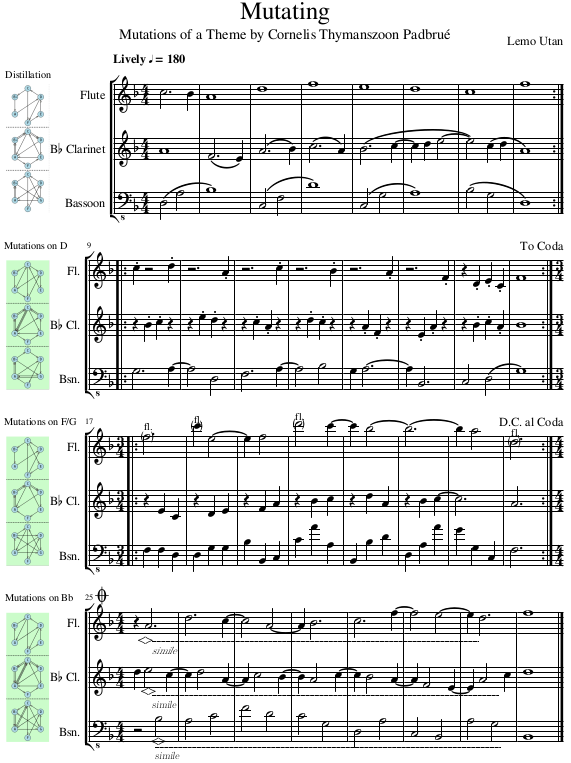Naturally one need not stop at one mutation, nor need one mutate on the same pitch, simultaneously, for each line. As long as one does not mutate a quiver on the same pitch twice (which simply takes you back to where you were) the mutations are - in general - endless (but not for the simple 14 state explanatory model presented earlier). In this particular example, we're always going to be in F major (or D minor, or E Locrian, G Dorian etc), but clearly 'seed' material from more chromatic pieces will tend to stay chromatic.

Note that there's no guarantee that, after each mutation, a full 'all-arrow-consuming' traversal is possible across a quiver one ends up with. But if they do exist then the more often one mutates, the more such possible traversals will exist and the longer will they become. In any case, there's no law forcing you to use only mutations which result in fully traversible quivers.

## 20200913

### Wedding Weeding

On a bright cold day in February of MDCXLII (though we doubt the clocks were striking XIII), Matthias Steyn and Marie van Napels got married in Haarlem.

Here's the first section of Cornelis Thymanszoon Padbrué's Synphonia in nuptias written for the occasion.Each of the three lines is a sequence of single notes and can be written as a path without regard to their length or octave. The first line is C, D, E, F, E, D, C♯, D, E, F, G, F, E, etc and it can be represented as a directed graph:where the arrows represent the transitions between consecutive notes in the melodic line, and the numbers on the arrows indicate how many times such a transition happens within it.

We're now ready to play a game with this graph.

## La Règle du jeu

• Remove all unicycles and bicycles
• Remove any resulting isolated notes

All of which sounds distressingly bike-unfriendly, notwithstanding an evident tricycle tolerance, but these are technical terms.

A unicycle is an arrow leaving a note and going right back to it without any intervening notes. This particular line has none, so there's nothing to do here. But Cantus II has a unicycle at bar 13 where a C is 'restarted' in bar 14. The Bass line is, like the first, devoid of unicycles.

A bicycle is an arrow from one note to another where there's also an arrow from that second note back to the first. There's an obvious one on the left of the above figure between G and A and also on the right between D and C♯.

Where there are multiple arrows in both directions between a pair of notes, we simply cancel opposite pairs and any that remain - perforce in only one direction - are retained. Thus the single arrow from A to B♭ will cancel one of the two from B♭ to A, leaving only one arrow from B♭ to A. Thus we end up with:Notice that we've completely lost two notes, C♯ and G. This is because both these notes were attached to each of their immediate neighbours by completely cancelling bicycles.

Note also that we've lost the numbers on the arrows. This is because bicycle cancellations - mostly - leave only one arrow which we shan't bother labelling with a 1. If two (or more) arrows remain, perforce in the same direction from one note to another, we shall draw them in explicitly - similarly unlabelled.

In fact this occurs in both of the other lines which we shall now process. First, Cantus II: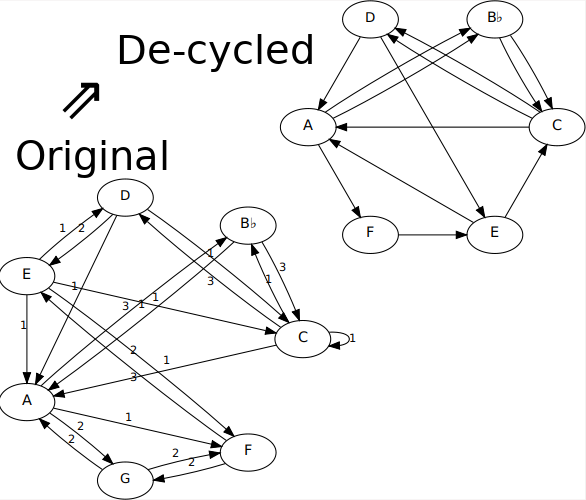where, for example, the unicycle at C has been removed and one of the three transitions from B♭ to C has been cancelled by the lone transition from C to B♭, leaving the two arrows shown. Notice again that G has been banished since it was connected exclusively by cancelling bicycles.

Finally, we'll go straight to the de-cycled version of the bass line: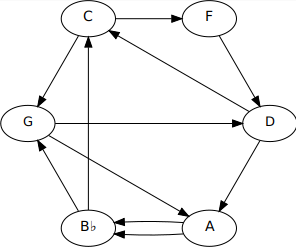## New Paths for Old

Obviously it's possible to find a path through each of the original graphs since that's how they were constructed in the first place.

The question is,

• Is it possible to find a path through each reduced graph, each arrow being used exactly once?
• You're allowed to start wherever you like. Let's reproduce the three graphs together here:Inspection shows a path C, B♭, A, D, C, F, E, D, F across the first line. It's 9 notes long and consumes all 8 arrows.

Musical considerations (the original is in either F major or D minor [OK so it might be B♭ Lydian, etc, too]) indicate that since we're beginning the first line with a C that we might consider the piece as being in F major and that we start with an A in line 2 and F in line 3 (or vice versa).

For line 2 we can find a path (of all 13 arrows) A, F, E, C, A, B♭, C, D, E, A, B♭, C, D, A.

And for line 3 we can find F, D, C, G, A, B♭, G, D, A, B♭, C, F, a path with 12 notes consuming all 11 arrows.

This is convenient - it also ends in F major, albeit sans its 5th. It's beginning to look like we can get a half-decent 8 bar sequence out of this. For example:And here's another one:If your browser supports it, these two chunks of pressed Padbrué may be heard (twice each) with the player below.

These are two (re-)compositions from the same three paths, and it's clear that - musically - there's no real limit to expressions of such 'distillations' although they'll probably all sound a bit 'samey'.

But is there a limit to the number of paths through any of these note-gardens? Are these the only paths? Can you find any others?

One is tempted to believe that - given their construction from a guaranteed initial path - there will always be at least one path in the reduction. But the pruning is pretty brutal - we can, after all, lose notes - so have we just been lucky with these three examples?

## Acknowledgements

By the Way, thanks to
• musescore for music composition, pics and midis
• mftext for converting midi to text
• awk for turning text into graphviz dot files
• graphviz for the graph-drawing

All free!

## 20200422

### The results are in

As promised in the last post, we have now calculated the lengths of aluminium tubing (of a certain grade) required to produce a set of tubular chimes - and even that is much too grandiose a term for the rather toy-like set of factory-cut hollow rods purchased, at the very reasonable price of about thirty quid (including delivery), for the experiment.

The material arrived the next day, and I measured the lengths principally because I needed accurate data for my calculations below - and only secondarily to check that they were as ordered (which they pretty much were, and certainly within the vendor's advertised tolerance). I also weighed them, principally to check that the density matched the theoretical density used in the frequency calculations - which of course it did, to within about a half of a percent. As I have no machinery to check its Young's modulus of elasticity, I continue to rely on its wikipedially reported value.

Measuring their frequencies was the most difficult task, and involved suspending the tubes with a hot-glued thread connecting the top of each tube to a shelf, and recording them - with my trusty H2N Zoom recorder - being lightly struck by a similar aluminium tube. Here's a composite of the noises made by this 'instrument'.

Do not be fooled by the levels. Although the samples in this recording are untreated (apart from being chopped up into segments), those small cylinders cannot shift very much air and the sounds are quite quiet. It's the H2N being held (by hand) up close.

The samples were brought into Cockos Reaper whereupon the use of its built-in band-pass filter, ReaEQ, (to cut out the highly audible harmonics) and frequency counter (ReaTune) plugins proved adequate to the task. Here are all the measurements, plus a picture of the set of tubes.

lengthweightfrequency$\kappa$13 Pipelings
($mm$)($gm$)(Hz)($m^2\,s^{-1}$)(png)
25110.6351432.4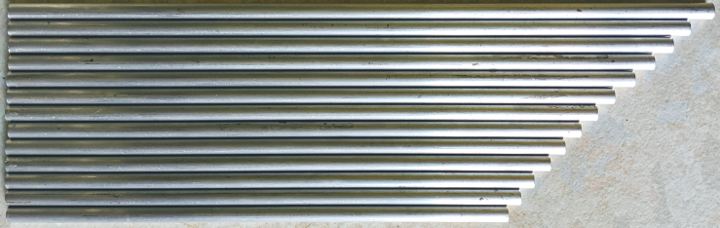24210.2355132.3
2369.9657932.2
2299.7562032.5
2229.4365132.1
2159.1569832.3
2108.8173432.4
2038.678632.4
1988.3282132.2
1938.1486632.3
1877.8792032.2
1827.6997032.1
1777.51101831.9

As expected, the frequencies were not even close to the design targets (respectively 165, 175, 185, 196, 208, 220, 233, 247, 262, 277, 294, 311, 330) and were - on average - 3.14 times higher (to the three significant figures employed throughout this exercise). The final column in the above table is $\kappa$, the diffusivity mentioned in the previous post, and is calculated as $L^2 \times f$ for each row ($L$ being the metre value). The average value for $\kappa$ is 32.2, the same multiple (3.14, weirdly close to $\pi$) of the value of 10.2 expected.

But - and also as expected - the instrument does indeed play a very serviceable chromatic scale. It may not start on the 165 Hertz concert pitch E (as requested) but instead a rather higher 514 Hertz non-concert pitch flat C, not quite a quarter tone below the 'proper' one. That's quite a way out, but our measured value of $\kappa$ could now be used to calculate a new series of $L$ values from $L=\sqrt{\kappa/f}$ - provided of course we were to use exactly the same material. All lengths would, accordingly, be multiplied by a factor of 1.77 (the square root of 3.14).

For this particular kind of aluminium alloy the previous post shows that $\kappa = 1420 \sqrt{D_o^2 + D_i^2}$, where $D_o = 0.006 m$ (i.e. 6mm) and $D_i = 0.004 m$ (i.e. 4mm). It's perfectly reasonable to incorporate the 'blame' for the (fudge) factor of 3.14 into the 'material constant' of 1420 and claim that in practice this constant should be 4450. This leaves us free to use larger inner and outer diameters as long as it's the same alloy to allow us to shift more air and make louder instruments. For example, if we have our heart set on a more robust 165 Hertz concert pitch E, we choose a larger diameter tubing (say half an inch with a 6.2mm bore). This would give us a new value for $\kappa$ - based on $D_o = 0.0127\,m$ and $D_i=0.0062\,m$ - of $4450 \times 0.01413 = 63$. From this we'd calculate a length of 618 mm.

And so on

### Fudge

So why was the calculation incorrect? Why would we need to use 4450 rather than 1420? As the 1420 was itself calculated - as $(22.4/8\pi) \sqrt{E/\rho}$ - from easily verifiable physical properties of a particular alloy of aluminium, the only places where the discrepancy could occur are in the value of 22.4 and from the way that $I$ was defined (in terms of $D_o$ and $D_i$). Perhaps an engineer can let me know where I went wrong?

Finally, here's a plot of frequency versus length of the tubes received in the mail. The least squares fit (from LibreCalc) is $f = 33.6 \times L^{-1.97}$, satisfyingly close to the model of $L^2 f = \kappa$ (with my measured average of $\kappa=32.2$).

## 20200420

### Amateur Hour

Overtaken by a sudden urge to manufacture a cheap musical instrument, this blogger was minded to research the solutions to the wave equation in cylindrical coordinates, with a view to constructing a set of tubular chimes ('bell' seeming a little grandiose a term for the small scale anticipated).

At the outset I would urge anyone in search of a real instrument to go to a professional (such as Matt Nolan, but do search around for others) because I have zero confidence that what I will receive will be anywhere near the frequencies I've specified, although I do expect them to be in tune(ish) relative to each other. We'll get to the reasons why soon enough - it's certainly not due to the metal merchant but to me, the utter amateur.

### Pragmatics

Engineers have already collapsed the mathematics into just the amount necessary for the problem at hand - typically the determination of the resonant frequency, $f$, of a pipe made out of a material of known elasticity and density. Consequently we can start with a formula such as is provided by Ron Frend

\begin{equation} f = \frac{1}{2\pi} \cdot 22.4 \cdot \sqrt{\frac{EI}{\mu L^4}} \end{equation}

where we know things like

• $E$ is the Young's Modulus of Elasticity
• $I$ is the 4th Polar Moment of Inertia
• $\mu$ is the Mass per unit length
The formula requires that we use $\mu$ as mass per unit length. The kind of tubing we can get from suppliers is typically specified with an inner and outer diameter, and so the area of the material in the pipe's cross-section is $\pi \cdot (D_o^2 - D_i^2) / 4$, giving us the required $\mu = \pi\rho \cdot (D_o^2 - D_i^2) / 4$, where $\rho$ is the material's density.

Our source provides a formula, $I=0.049 \times(D_o^4 - D_i^4)$. This is the first place where I encounter a problem since other authorities give $I=\pi(D_o^4 - D_i^4)/32$ which evaluates to twice the value of the one provided here (and by others - he's by no means alone) since $0.049 = \pi / 64$.

It's why I have little confidence that I'm going to get the frequencies I imagine I'm asking for. Regardless, we may reformulate - taking this additional information into account and switching the dependency of $f$ upon $L$ to that of $L$ upon $f$:

\begin{equation} \begin{split} L^2 &= \frac{1}{2\pi} \cdot \frac{22.4}{f} \cdot \sqrt{\frac{\pi E \cdot (D_o^4 - D_i^4) \cdot 4}{\pi\rho \cdot (D_o^2 - D_i^2) \cdot 64}}\\ &= \frac{22.4}{8 \pi f} \cdot \sqrt{\frac{E}{\rho} (D_o^2 + D_i^2)} \end{split} \end{equation}

For a typical grade 6063 Aluminium sold by commercial metal merchants, we use $E = 6.83\times 10^9\,kg\,m^{-1}s^{-2}$ and $\rho = 2690\,kg\,m^{-3}$ and employ the three significant digit formula:

\begin{equation} L^2 \approx \frac{1420}{f}\sqrt{D_o^2 + D_i^2} \end{equation}

Consequently, this adventurer has ordered the following cuts of 4mm inner diameter and 6mm outer diameter with an intent to construct a full chromatic scale

f (Hz)L (mm)
165 249
175241
185235
196228
208221
220215
233209
247203
262197
277192
294186
311181
330176

As stated, we don't expect the frequencies to be correct - principally due to some conflicting information about the formulæ found online. The pipe vendor guarantees only a cut accuracy of ±2mm. (The formula indicates a discrepancy of 2% in $f$ for every 1% discrepancy in $L$). However, even if the 'base' frequency is way off, we'd expect the entire system to be mostly in tune with itself since the frequency ratios should still be chromatic if the length ratios are as directed.

When the material arrives, we shall measure the actual lengths and frequencies and see how far off we are. As an experiment (even if dire failure frequency-wise) it should still give us a constant value $\kappa$ - a kind of diffusivity - for this particular material which we can use in a formula $L^2 f = \kappa$ to produce a more accurate set, possibly in an exotic scale of 19 TET with a complement of 20 tubes.

My ineptitude may be amusing. Watch this space.

## 20200228

### Symmetries

The symmetries of the dodecagon, the regular 12-sided polygon used in models of Pitch Class Set Theory, are well known. Viewed as a dihedral group (of order 24), it has a class index

$$P_{D_{12}}(\textbf{x}) = \frac{1}{24} \left( x_1^{12} + 7 x_2^6 + 2 x_3^4 + 2 x_4^3 + 2 x_6^2 + 4 x_{12} + 6 x_1^2 x_2^5 \right)$$

which sheds some light upon the 2, 3, 4 and 6-fold symmetries of 12-sided figures. This can be used to enumerate all the essentially different (equivalent under rotations and reflections) shapes of all (mostly) irregular polygons of all orders - from 0 to 12 - inscribable within it. One simply replaces every $x_i$ with $(1 + t^i)$ in the above (Pólya Enumeration) to recover the following degree 12 polynomial in t:

$$E_{12}(t) = 1 + t + 6 t^2 + 12 t^3 + 29 t^4 + 38 t^5 + 50 t^6 + 38 t^7 + 29 t^8 + 12 t^9 + 6 t^{10} + t^{11} + t^{12}$$

which counts the number of essentially different k-gonal shapes - as the coefficient of tk - corresponding to all 224 Pitch Class Sets of different sizes available to Twelve Tone Music.

If you count non-symmetric shapes twice (i.e. as distinct from their reflections) rather than just once, the Cyclic Group polynomial enumerator - $1 + t + 6 t^2 + 19 t^3 + 43 t^4 + 66 t^5 + 80 t^6 + 66 t^7 + 43 t^8 + 19 t^9 + 6 t^{10} + t^{11} + t^{12}$ - is the appropriate one to use. This aggregates 352 distinct shapes (evaluate the polynomial at t = 1). Because it counts mirror-pairs (musically inverted PC sets) as distinct, this enumeration reveals that there are (352 - 224) = 128 pairs of non-symmetric shapes and 352 - 2 × 128 = 96 symmetric ones.

So far, so standard (this blog also catalogues them all here).

Most of these tk coefficients are rather haphazard (like, say, 29 and 38) and have no particularly friendly relations with the polygon they inhabit - rather to be expected. However, we see that the number of 3-sided shapes is 12, a number very friendly indeed to the enclosing dodecagon. It means that there's a possibility that all the different 3-sided shapes - and here they all are - might be enticed to exactly fit, four at a time, into three separate dodecagons.

### Set Coverings

Note that the coefficient 6 (of t2) is also '12 friendly'. But tilt and move them as you will, it's impossible to fit the 6 different 'diangles' into one dodecagon, so that each occupies a different dodecagonal vertex pair. It's not possible to cover (or partition) the set {1, 2, 3, 4, 5, 6, 7, 8, 9, 10, 11, 12} with 6 non-intersecting 2-sets where each such 2-set represents each of the six possible differences (on the dodecagon, i.e. modulo 12 differences) between the 12-set's points.

### Octatonality

If we drop back to a tonality in which only 8 Pitch Classes are available, the Class Index of this system is

$$P_{D_{8}}(\textbf{x}) = \frac{1}{16} \left( x_1^8 + 4 x_1^2 x_2^3 + 5 x_2^4 + 2 x_4^2 + 4 x_8 \right)$$

we find its catalogue of (30) essentially different sub-polygons is enumerated by

$$E_{8}(t) = 1 + t + 4 t^2 + 5 t^3 + 8 t^4 + 5 t^5 + 4 t^6 + t^7 + t^8$$

One notices that the t2 term has coefficient 4, indicating that octatonicity's 4 interval classes 1, 2, 3 and 4, might be capable of covering itself in a single bound. And indeed it is. Interval class 1 can cover pitch classes 2, 3 and (switching to abbreviations) IC 2 can cover PCs 6, 8, IC 3 can cover PCs 4, 7 and finally IC 4 can cover PCs 1, 5. It's the only way of doing it with regard to equivalence of rotational and reflective symmetries.

But as a musical transformer - say, as the PC permuter (1,5)(2,3)(4,7)(6,8) - it's not very interesting since all it can do is exchange pitch classes. As such a transformation is an interval-preserving operation, it's pretty much a do-nothing, musically speaking. This is why, in general, we don't regard any tonality covers with PC Sets containing fewer than 3 pitch classes as interesting

But we're not quite done yet with the octagon since there's another friendly coefficient in the 8t4 which indicates that we may have a quadruple covering of the octagon with its four pairs of tetragons (possibly more commonly referred to as quadrilaterals). However such hopes are quickly dashed since one of the 8 quadrilaterals must be a square, and placing a square inside an octagon requires a second square to complete the cover, thus violating our règle du jeu that each shape be used exactly once. We don't even need to bother with the other shapes as we're already dead in the water.

This is a general upper limit to the size of an 'inner polygon'. Once we reach the halfway point of an evenly sized polygon, a subset of exactly half the size which covers every other vertex can complete a cover only with a duplicate of itself to soak up the remaining 'every-other' vertices. Beyond the halfway point of course, there's no possibility of coverage since too few vertices remain.

Our mission, then, is to find the 'special' values of n and k (2 < k < n/2) where rotationally and reflectionally equivalent binary colourings of n-gons (creatures known as necklaces) carry Cn,k (generally irregular) k-sized sub-polygons. We require that k divides both n (permitting n-set coverings with p = n/k of its k-sized subsets) and Cn,k itself (permitting use of all equivalent k-gons) and where p divides Cn,k (permitting complete coverage of Cn,k/p separate n-gons).

### The Triumph of the Dodecagon

And so, by this definition, the first polygon where there's even a possibility that a complete set of dihedrally equivalent Pitch Set Classes will be capable of covering its own tonality is the dodecatonic. It's the first one large enough.

It's reasonably obvious that tonalities with a prime number of pitch classes don't even get a look-in with regard to subset covering. So goodbye to 17TET, 19TET, 31TET etc. The 15TET, non-prime, system is quite popular but none of its subset shape collections occur in quantities appropriate to such coverages. The only candidates for tonalities up to 64TET are presented here:

### Coverings and Applications

The power of the enumerative polynomial lies in its proof of existence of such potential coverings. But it's non-constructive and cannot tell us how to build the things it counts. Still less does it tell us anything about their set-covering capabilities.

So, given the unlikelihood of coverage indicated by the previously shown impossibility of 'dianglising a dodecagon' (in musical terms, 'interval-covering a chromatic scale'), and despite the very real lack of interest we have in covering polygons with anything smaller than triangles in any case, the big question is can all 12 triangles (musical triads) - taken four at a time - fit into three dodecagons so that none of their vertices occupy the same dodecagonal vertex (musical pitch class) as another's?

Covering the first dodecagon is easy since there's so much choice. Even with only 8 triangles left, a second cover remains only mildly troublesome. But with only four remaining triangles, a final cover is hard to find since there are so many ways to rotate and reflect them. By the time you have four left, if your earlier choices are unsuitable then the final cover will likely be impossible.

But there are many solutions. Thousands, in fact. Here is one.

In fact this particular solution is not found by trial-and-error but is rather special even in terms of the game itself. We've used the computer algebra system, GAP, to find solutions. Of the thousands of solutions, this is one from a tiny subset of 20 of them. All of the others, except one, which is really special, are of the same class.

The vast majority of triple-set coverings by triad quadruples are found as an example of the Alternating Group on 12 symbols (usually notated as A12). This is a simple group of order 12!/2 = 239500800. Despite the term 'simple' (a term of Group Theory meaning that it has a simple structure and is akin to a prime number in Number Theory) the way this group can fling things about is prodigious, as the number of its operations suggests.

But the configuration above is an example of an arguably more interesting group discovered in the 19th century. It's one of the sporadic simple groups, specifically the Mathieu Group M12. But it is somewhat less flingy as its order is a paltry 95040. And from an applicative point of view it's often nicer to have fewer choices (the apparently paradoxical idea behind 'constraint sets you free') since it's easier to actually make a beginning.

The way the group features into these arrangements is by way of construction. If we take the first of the three coverings we can use it as a permutation - specifically (1,2,3)(4,5,7)(6,9,11)(8,10,12), the four clockwise tricycles in the covering - to generate a group. If we regard the numbers as pitch classes (it's usually 0 to 11 in musical PC Set theory but we can equivalence 12 and 0 with impunity) then we may operate upon any musical segment (the choice of what constitutes a segment is completely up to the applier) by permuting its pitch classes with that permutation. Each pitch class moves (conventionally clockwise) around the triad it finds itself on. For example we might have a segment with pitch classes { 1, 4, 8, 11 } - which could represent a $C\sharp m7$ chord. The aforementioned permutation moves 1 to 2, 4 to 5, 8 to 10 and 11 to 6 (the wraparound) and thereby produces the new set { 2, 5, 10, 6 } - re-presented as { 2, 5, 6, 10 }. This set may - if interpreted as rooted on pitch class 6 (PC Set theory conventionally $F\sharp$) - be perceived as $F\sharp^{+} Maj 7$.

Such a transformation may be applied to any musical segment, such as the one shown below.

A second use of the same permutation would then transform the PC Set from { 2, 5, 6, 10 } to { 3, 7, 9, 12 } (perhaps $Am7\flat5$?). By the way, that's 3 of the seven sevenths, so this - admittedly tiny - orbit fixes some kind of seventhiness, if you will.

And a third application will of course return us to the initial set, since all cycles are of the same size, i.e. the order of the permutation is 3. This single permutation has a class index of $x_3^4$ (being a product of 4 3-cycles) and the group it generates is the tiny simple cyclic group of order 3, C3.

The Group M12 is generated when all three coverings are used to construct permutations. The other two are - respectively from the above figure - (1,4,7)(2,5,9)(3,8,10)(6,11,12) and (1,5,9)(2,3,6)(4,8,10)(7,11,12) and although each is a simple $x_3^4$ cycle, when allowed to operate together by composition the group of permutations has the class index

\begin{split} P_{M_{12}}(\textbf{x}) = \frac{1}{95040} ( x_1^{12} + 495 x_1^4 x_2^4 &+ 2970 x_1^4 x_4^2 + 1760 x_1^3 x_3^3 + 396 x_2^6 + 11880 x_1^2 x_2 x_8\\ &+ 9504 x_1^2 x_5^2 + 15840 x_1 x_2 x_3 x_6 + 2970 x_2^2 x_4^2 + 2640 x_3^4 + 17280 x_1 x_{11}\\ &+ 9504 x_2 x_{10} + 11880 x_4 x_8 + 7920 x_6^2 ) \end{split}

If we label these three permutations with the letters 'a', 'd', and 'm' - for no particular reason - then the successive application of, say m followed by a followed by d would be written as mad. It would be the permutation (2,4,3)(5,12,7,11,10,9)(6,8), which is one of the $15840 x_1 x_2 x_3 x_6$ permutations with that particular shape (the $x_1$ in this case representing the point 1, or pitch class $C\sharp$, which happens to be fixed by this particular permutation).

If we apply this mad operation to a fairly well-known melodic segment, we obtain the following:

To recover the original, one would apply the inverse operation $(mad)^{-1} = d^{-1}a^{-1}m^{-1}$ - which is of course the 'undoing' permutation (2,3,4)(5,9,10,11,7,12)(6,8). Because such transformations operate only upon pitch classes however, there can be no indication of which particular octave the original pitch class was in and such information is lost.
There are also relationships between the group's generators. One such relation is found when we look at dm-1 = (1,10,2)(3,4,12)(6,7,9), one of the $1760 x_1^3 x_3^3$ which - in this case - fixes the triad {5, 8, 11}. Since this is clearly an operation of order 3 (comprising exclusively 3-cycles) then any set acted upon by it thrice will reappear. This means that dm-1dm-1dm-1 = (dm-1)3 = (), the group's identity operation. It's also (dm2)3 because the square of any of the generators is also the generator's inverse (two clockwise turns gets you to the same place as one anticlockwise turn).
As we're on the subject of the Mathieu Group M12, Dave Benson's book mentions that Messiaen's piano piece Ile de Feu 2 uses the 2 permutations (1,7,10,2,6,4,5,9,11,12)(3,8) and (1,6,9,2,7,3,5,4,8,10,11) to transform both tones and durations. The first permutation being one of the $9504 x_2 x_{10}$ and the second one of the $17280 x_1 x_{11}$, they are themselves nothing directly to do with dodecatonic coverages by triads. It's extremely unlikely that the particular triplet of generators we're using here (out of the 20 possible coverages we've found) has any correspondence, pitch-class-wise, with Messiaen's. But it is nonetheless possible to write them in terms of ours, the first as $d a^2 d^2 m a d^2 m^2 a d m^2 (a^2 d)^2 a m d m^2 a^2 d^2 a m^2$ and the second as the slightly simpler (!) $m^2 d^2 (m a^2 d)^2 a^2 d$. These are also calculated by GAP, but such permutation re-mappings are quite tough to work out and it's possible that there are shorter paths from our madness to Messiaen.